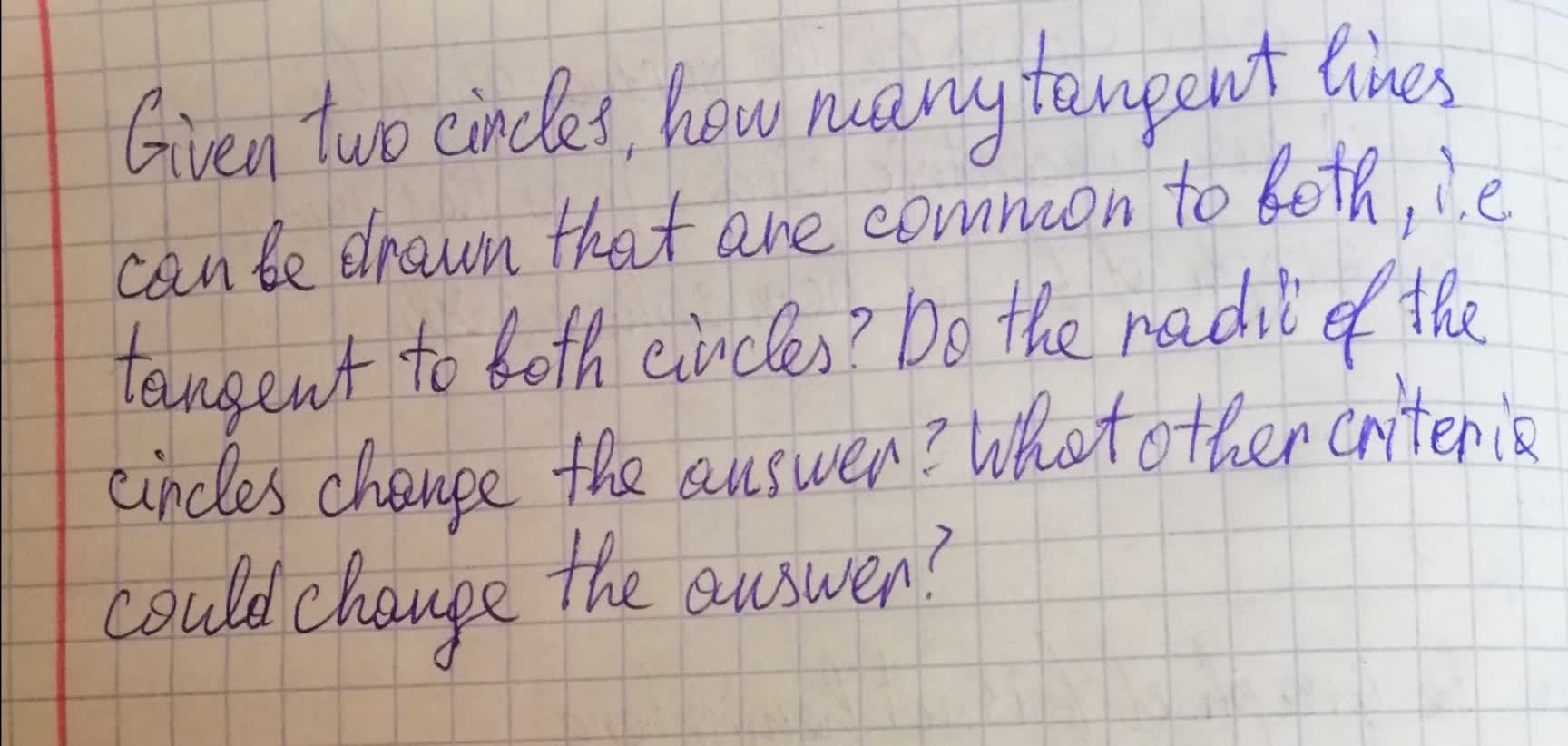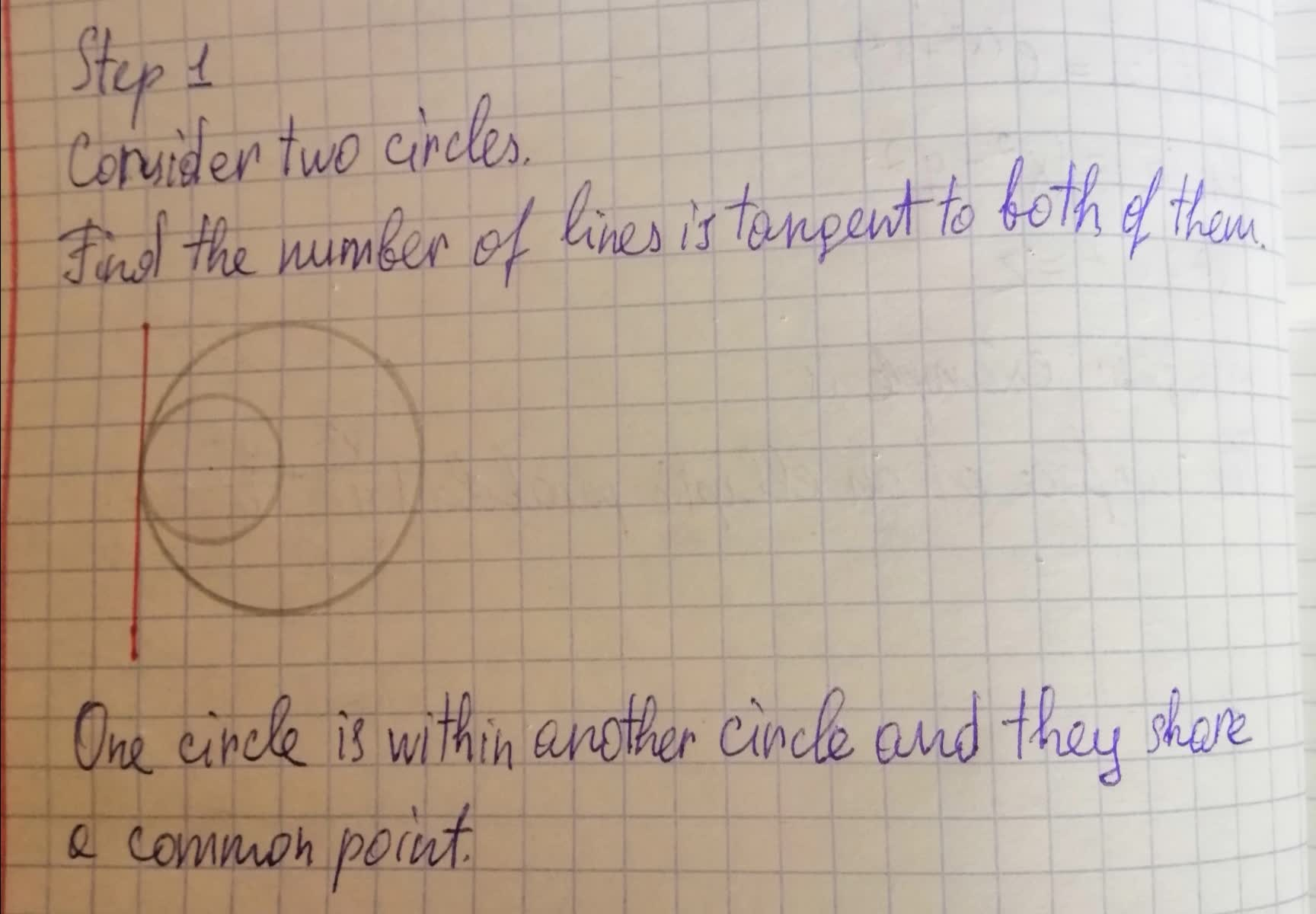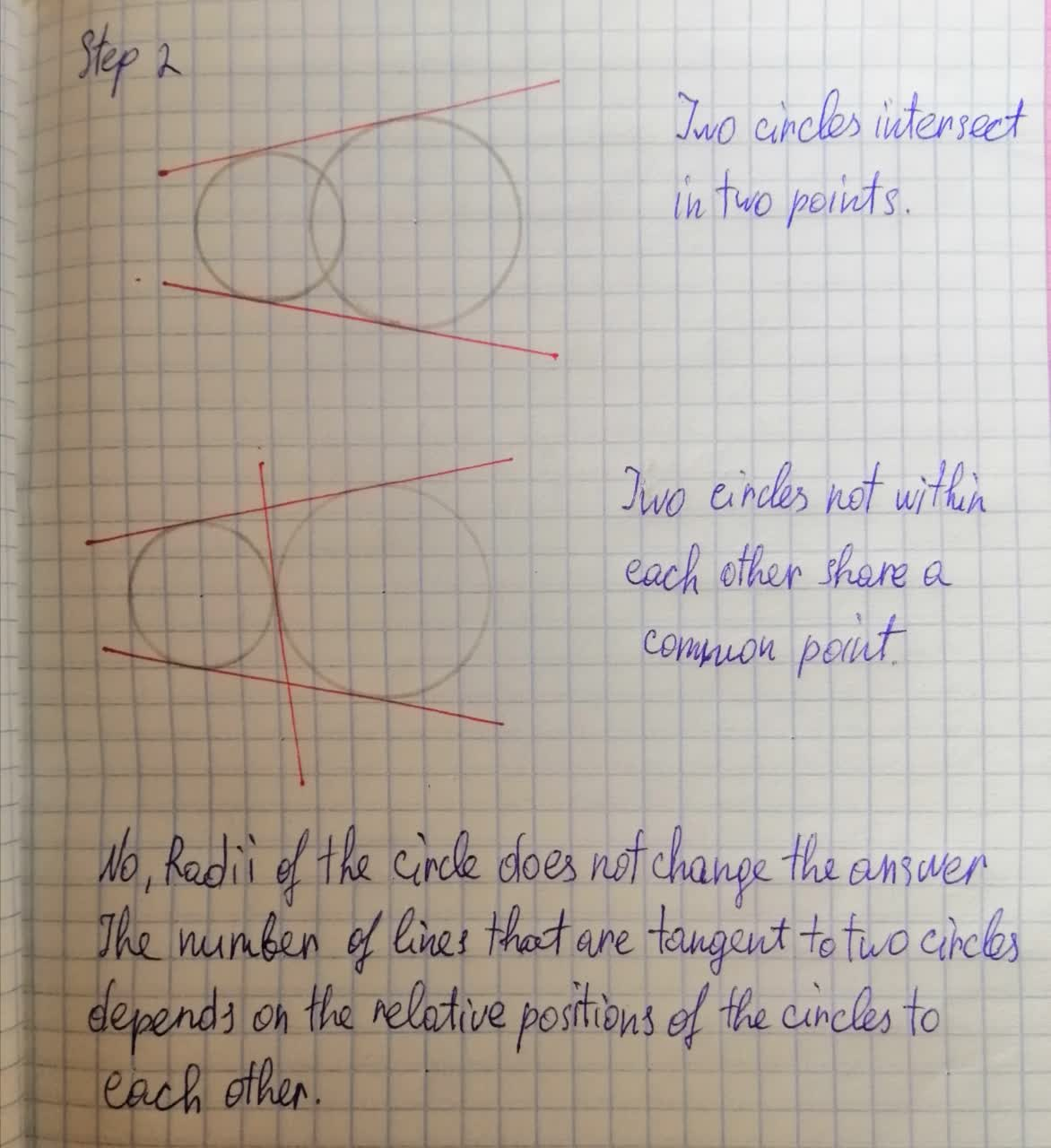Given two circles, how many tangent lines can be drawn that are common to both, i.e. tangent to both circles? Do the radii of the circles change the answer? What other criteria could change the answer?Kye 2021-08-12 Answered
Given two circles, how many tangent lines can be drawn that are common to both, i.e. tangent to both circles? Do the radii of the circles change the answer? What other criteria could change the answer?• Questions are typically answered in as fast as 30 minutes

Solve your problem for the price of one coffee

• Math expert for every subject
• Pay only if we can solve itLeonard Stokes

Given the condition of two circles, we can give 3 examples of their location